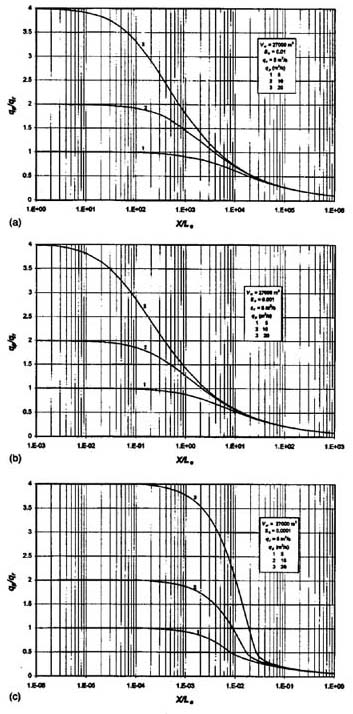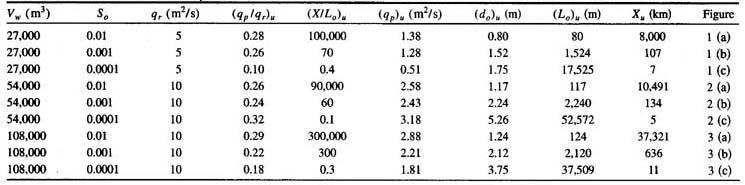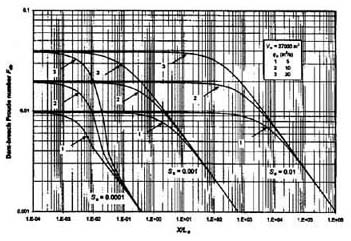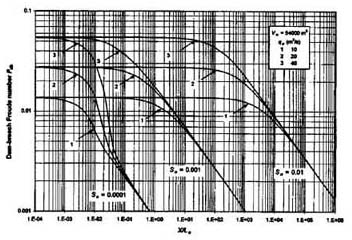Dam-Breach Flood Wave Propagation Using Dimensionless Parameters

Victor M. Ponce, M. ASCE; Ahmad Taher-shamsi; and Ampar V. Shetty

Online version 2018

[Original version 2003]

 ABSTRACT An analytical model of flood wave propagation is used to study the sensitivity of dam-breach flood waves to breach-outflow hydrograph volume, peak discharge, and downstream-channel bed slope. Dimensionless parameters are identified for discharge and distance along the channel. A dam-breach Froude number is defined to enable analysis through a wide range of site and flow conditions. It is found that, at a certain distance downstream, the attenuated peak discharge is independent of the magnitude of the discharge at the breach site. This attenuated peak discharge is termed the "ultimate discharge," and the distance associated with it, the "ultimate distance." These ultimate values are a function, primarily of the downstream-channel bed slope and, secondarily, of the breach-outflow hydrograph volume.

1.  INTRODUCTION

Flash floods occur with little warning and may result in considerable damage to life and property. They can be caused by cloudbursts or sudden releases from dam breaches. A dam-breach flood wave propagates along a river reach with velocity and depth usually decreasing with time and distance. Forecasting dam-breach flood waves has received considerable attention in the literature (Fread 1988; Singh 1996).

The breach-outflow hydrograph is a function of the geometric and hydraulic properties of the reservoir and of the geotechnical characteristics of the embankment. Its determination is usually subject to great uncertainty. From a practical standpoint, given a feasible range of breach-outflow hydrographs at a site, there is a need to evaluate the propagation of these flood waves.

For any given reservoir, the dam-breach flood peak is inversely related to the flood duration and, by extension, to the time-of-rise of the outflow hydrograph. Since the flood wave attenuation is inversely related to the time-of-rise (Ponce et al 1978; Ponce 1989), it follows that several postulated breach-outflow hydrographs at a site may eventually attenuate to about the same peak discharge. This fact has been experimentally confirmed in the literature (Chen and Armbruster 1980; Petrascheck and Sydler 1984).

This paper uses an analytical model of flood wave propagation (Ponce and Simons 1977) to study the sensitivity of dam-breach flood waves to a postulated range of breach-outflow hydrographs. The objective is to show, using dimensionless parameters, that a flood stage resulting from a dam-breach failure becomes eventually, i.e., at a certain distance downstream, independent of the magnitude of the peak discharge at the breach site.

2.  THEORETICAL BACKGROUND

Beginning with Saint Venant's early work (Saint Venant 1848; 1871a,b), several investigators have studied the phenomena of propagation of shallow waves in open-channel flow. In the past century, a substantial body of knowledge has been developed to describe one-dimensional flood propagation (Seddon 1900; Thomas 1934; Hayami 1951; Lighthill and Whitham 1955; Stoker 1957; Ponce and Simons 1977).

Analytical studies of dam-breach flood wave propagation must include all relevant forces, i.e., gravity, friction, pressure gradient, and inertia. When all these forces are taken into account, the result is a set of two partial differential equations of water continuity and motion (Liggett 1975; Liggett and Cunge 1975). While there is a number of analytical solutions for these equations (Lighthill and Whitham 1955; Dooge 1973; Ponce and Simons 1977), no complete analytical solution is available to date. The linear solution of Ponce and Simons (1977) is significant because it provides a good insight into the various types of shallow water waves, including kinematic, diffusion, dynamic, and gravity waves.

The equations of gradually varied unsteady flow in a prismatic channel of a rectangular cross section, expressed in terms of unit width, are (Liggett 1975):

Equation of continuity

 ∂d            ∂u           ∂d_____  + d ____  + u ____  = 0  ∂t             ∂x           ∂x (1)

and equation of motion

 1       ∂u            u       ∂u           ∂d            ____  _____  +   ____  _____  +   _____  +   Sƒ  - So  =  0   g       ∂t            g       ∂x            ∂x (2)

in which u = mean velocity; d = flow depth; g = gravitational acceleration; Sƒ = friction slope; So = bottom slope; x = space; and t = time.

In uniform flow, Sƒ = So, and Sƒ is related to the bottom shear stress τo as follows (Chow 1959):

 τo            Sƒ  =  ______             γdo (3)

in which γ = density of water; and do = equilibrium flow depth.

The perturbation equations corresponding to Eqs. (1) and (2) are, respectively (Ponce and Simons 1977):

 ∂d'             ∂u'              ∂d'_____  + do _____  + uo _____  = 0  ∂t               ∂x               ∂x (4)

 1       ∂u'           uo     ∂u'           ∂d'                 τ'        d' ____  _____  +   ____  _____  +   _____  + So ( ____  - ____ )  =  0   g       ∂t            g       ∂x            ∂x                 τo       do (5)

in which, generally, a variable ƒ has been expressed as ƒ = ƒo + ƒ' ; with ƒo = equilibrium value; and ƒ' = small perturbation. In order to make Eqs. (4) and (5) mathematically tractable, the bottom shear stress is related to the mean velocity as follows:

 1            τ  =  ___ ƒρu 2          8 (6)

in which ƒ = Darcy-Weisbach friction factor and ρ = mass density of water. Using Eq. (6), Eq (5) reduces to:

 1       ∂u'           uo     ∂u'           ∂d'                  u'       d' ____  _____  +   ____  _____  +   _____  + So ( 2 ___  - ___ )  =  0   g       ∂t            g       ∂x            ∂x                   uo      do (7)

The transformation of Eqs. (4) and (7) to the frequency domain is accomplished by seeking a solution in sinusoidal form (Ponce and Simons 1977), such that:

 d'            ____ = d* exp [i (σ* x* - β* t*)]  do (8)

 u'            ____ = u* exp [i (σ* x* - β* t*)]  uo (9)

in which d* and u* = dimensionless depth and velocity amplitude functions, respectively; σ* = dimensionless wavenumber, defined as σ* = (2π/L)(do/So); and β* = complex propagation factor, with L = wavelength and i = ( -1 )1/2.

The substitution of Eqs. (8) and (9) into Eqs. (4) and (7) yields:

 Fo2 β*2 - 2 (σ*Fo2 - i )β* - [σ*2 ( 1- Fo2 ) + 3σ*i )] = 0 (10)

in which Fo = uo / ( gdo )1/2 = Froude number.

The solution of Eq. (10) is (Ponce and Simons 1977):

 1            β*  =  σ* ( 1 - i ζ ) + σ* [( _____ - ζ 2 ) + i ζ ]1/2                                           Fo2 (11)

in which:

 1            ζ  =  _______           σ*Fo2 (12)

In general, β* is a complex function expressed as follows:

 β* = βR + i βI (13)

 C + A     βR  = σ* [1 ± ( _______ )1/2 ]                              2 (14)

 C - A     βI  = -σ* [ζ ∓ ( _______ )1/2 ]                              2 (15)

in which:

 1            A  =  _____ - ζ 2           Fo2 (16)

 1            C  = [( _____ - ζ 2 )2 + ζ 2 ]1/2               Fo2 (17)

The following definitions apply: uo = steady equilibrium flow mean velocity; do = steady equilibrium flow depth; So = bed slope; L = wavelength of sinusoidal perturbation; T = wave period of sinusoidal perturbation; c = wave celerity; Lo = reference channel length, i.e., the length in which the steady equilibrium flow drops a head equal to its depth; σ* = dimensionless wavenumber; and τ* = dimensionless waveperiod, such that:

 L            c  =  ____            T (18)

 do            Lo  =  ____             So (19)

 2π         σ*  = ( ____ ) Lo              L (20)

 uo         τ*  = T ( ____ )                  Lo (21)

3.  MODEL DEVELOPMENT

Following Ponce and Simons (1977), the flood wave attenuation follows an exponential law in which the amplitude at a given time t is equal to the initial amplitude at to multiplied by eβ*lt*, in which t* = (t - to)uo / Lo. Therefore:

 qp = qpo e β*I t* (22)

such that:

 qp                                  C - A     _____  = exp { -σ* [ζ - ( _______ )1/2 ]t* }   qpo                                    2 (23)

For to = 0, t* = tuo /Lo. Assume the wave celerity c = ck = muo, in which ck is the kinematic wave celerity; then t = X / c = X / (muo), in which X is the distance along the river reach, from the dam-breach site to the point of interest. It follows that:

 qp       _____  = exp ( -α* X* )   qpo (24)

in which the discharge attenuation factor α* is:

 2 π                C - A     α*  =  _______ [ζ - ( _______ )1/2 ]            m 2τ*                 2 (25)

and the dimensionless distance is:

 X       X*  =  ____            Lo (26)

The wave attenuation is a function of dimensionless parameters, such that:

 qp           ____  = ƒ1( α* , X* )  qpo (27)

and

 α* = ƒ2 ( m, τ*, Fo, X* ) (28)

During the breaching of an earth dam, usually the total volume of water stored in the reservoir is released as a flood wave. Assuming a sinusoidal hydrograph shape for simplicity, the reservoir volume Vw is related to the flood-wave peak discharge Qp and hydrograph duration T as follows:

 1       Vw  =  __ QpT            2 (29)

or

 1       Vw  =  __ qpBT            2 (30)

in which qp = unit-width peak discharge and B = average width of the downstream river reach. The reference flow can be taken as

 1       qo  =  __ qp           2 (31)

 qo = uo do (32)

The substitution of Eqs. (30) to (32) in Eq. (25) leads to the following:

 2 π         Lo do B                C - A     α*  =  ______ (___________) [ζ - ( ______ )1/2 ]            m 2            Vw                       2 (33)

or, alternatively, using Eq. (19):

 2 π         do2 B               C - A    α*  =  ________ (_______) [ζ - ( _______ )1/2 ]            m 2 So        Vw                    2 (34)

4.  APPLICATION

The wave attenuation model represented by Eqs. (24)-(26) was applied to a series of hypothetical examples, varying breach-outflow hydrograph volume, downstream-channel bed slope, and unit-width peak discharge. The breach-outflow hydrograph volume Vw is the same as the reservoir volume at the time of the breach, expressed in terms of volume (m3) per unit of downstream channel width. The downstream-channel bed slope So is the equilibrium channel slope of the reach immediately below the damsite (for simplicity, assumed to be constant in this application). The unit-width peak discharge qp is the peak discharge calculated immediately below the damsite.

For the breach-outflow hydrograph volume, three sets of likely events were selected, encompassing the following breach durations: 0.75 h (short), 1.5 h (average), 3 h (long), and 6 h (very long); and unit-width peak discharges: 5 (low), 10 (average), 20 (high), and 40 m2s-1 (very high). Assuming sinusoidal breach hydrographs, the first volume [Eq. (30)] is: 0.5 (5 m2s-1 × 3 h) = 0.5 (10 m2s-1 × 1.5 h) = 0.5 (20 m2s-1 × 0.75 h) = 27,000 m3. The second volume is: 0.5 (10 m2s-1 × 3 h) = 0.5 (20 m2s-1 × 1.5 h) = 0.5 (40 m2s-1 × 0.75 h) = 54,000 m3. The third volume is: 0.5 (10 m2s-1 × 6 h) = 0.5 (20 m2s-1 × 3 h) = 0.5(40 m2s-1 × 1.5 h) = 108,000 m3.

Equation (30) was used to calculate the hydrograph duration (flood-wave period) T. Three downstream-reach bed slopes were chosen to encompass a wide range of values found in engineering practice: So = 0.01 (steep), 0.001 (average), and 0.0001 (mild). Values outside of these ranges are considered unusual.

The adopted value of m = 5/3 is applicable to Manning friction in hydraulically wide channels (Chow 1959). Manning's n = 0.05 was assumed; this value is judged to be in the midrange of typical field conditions (Barnes 1967).

Figures 1-3 show the results of dam-breach flood propagation calculations using Eqs. (24)-(26), for reservoir volumes of 27,000, 54,000, and 108,000 m3, respectively. Each figure has three parts: (a), (b), and (c), corresponding to bed slopes of 0.01, 0.001, and 0.0001, respectively. The examination of these figures shows conclusively that dam-breach flood waves eventually attenuate to the same peak discharge at a certain dimensionless distance downstream, regardless of the damsite peak discharge. This attenuated discharge is referred to as the "ultimate peak discharge" (qp)u and its associated dimensionless distance the "ultimate dimensionless distance" (X / Lo)u. Both are functions, primarily of bed slope and, secondarily, of hydrograph volume, as shown in Table 1.

An alternate way of analyzing the results of the dam-breach flood propagation model is accomplished by defining a dam-breach Froude number as follows:

 qp Fdb  =  __________             ( g Vw )0.5 (35)Fig. 1  Dimensionless peak discharge as function of dimensionless distance, for Vw = 27,000 m3,
and (a) So = 0.01; (b) So = 0.001; and (c) So = 0.0001.Fig. 2  Dimensionless peak discharge as function of dimensionless distance, for Vw = 54,000 m3,
and (a) So = 0.01; (b) So = 0.001; and (c) So = 0.0001.Fig. 2  Dimensionless peak discharge as function of dimensionless distance, for (a) Vw = 108,000 m3 and So = 0.01;
(b) Vw = 54,000 m3 and So = 0.001; and (c) Vw = 54,000 m3 and So = 0.0001.Table 1.  Ultimate peak discharge (qp)u and corresponding ultimate distance Xu for selected values of Vw and So.

Figures 4-6 show the results of flood propagation calculations using Eqs. (24)-(26), with dam-breach Froude number [Eq. (35)] plotted in the ordinate axis. Hydrograph volume, bed slope, and peak discharge are varied in the same manner as with Figs. 1-3. These figures generalize the behavior of dam-breach flood waves for a wide range of flow conditions.Fig. 4  Dam-breach Froude number as function of dimensionless distance, for Vw = 27,000 m3.Fig. 5  Dam-breach Froude number as function of dimensionless distance, for Vw = 54,000 m3.Fig. 6  Dam-breach Froude number as function of dimensionless distance, for Vw = 108,000 m3.

5.  CONCLUSIONS

An analytical model of one-dimensional unsteady open-channel flow has been used to study the propagation of flood waves following a postulated dam-breach failure, under a wide range of reservoir, breach and downstream flow conditions. A significant feature of the model is its ability to depict the flood wave travel and attenuation in terms of dimensionless parameters. The non-linear features of the phenomena are preserved by calculating reference hydraulic variables at short intervals throughout the simulation. The sensitivity of the routed flood wave to the magnitude of the breach peak discharge, a quantity whose determination is usually subject to great uncertainty, is determined.

It is found that dam-breach flood waves will attenuate to the same peak discharge at a certain distance downstream. This discharge and its associated distance are termed the "ultimate discharge" and the "ultimate distance", respectively. These values are a function, primarily of the bed slope and secondarily, of the hydrograph volume. In particular, the ultimate distance is strongly related to the bed slope, as shown in Table 1. It is confirmed that flood waves traveling in steep slopes (So = 0.01) have a tendency to be kinematic, i.e., to attenuate little throughout the propagation. Conversely, flood waves traveling in mild slopes (S0 = 0.0001) have a tendency to be very strongly diffusive (dynamic), i.e., to attenuate very fast. For instance, for Vw = 54,000 m3, So = 0.0001, and for dam-breach peak discharges in the range 10-40 m2/s, the ultimate peak discharge (qp)u = 3.18 m2s-1 and it occurs at a distance Xu = 5 km downstream of the damsite.

The findings of this study are useful in characterizing dam-breach flood waves through a wide range of site and flow conditions. This should be of assistance in the planning of programs of emergency preparedness and flood mitigation.

REFERENCES

1. Barnes, H. A. 1967. "Roughness characteristics of natural channels." U.S. Geological Survey Water-Supply Paper 1849, Washington, D.C.

2. Chen, C. L., and J. T. Armbruster. 1980. "Dam-break wave model: Formulation and verification." J. Hydraul. Div., Am. Soc. Civ. Eng., 106(5), 747-767.

3. Chow, V. T. 1959. Open-channel hydraulics. McGraw-Hill, New York.

4. Dooge, J. C. I. 1973. "Linear theory of hydrologic systems." Technical Bull. 1468, U.S. Dept. of Agriculture, Washington, D.C.

5. Fread, D. L. 1988. "BREACH: An erosion model for earthen dam failures." NWS Rep., National Oceanic and Atmospheric Administration, Silver Spring, MD, revised, 1991.

6. Hayami, S. 1951. "On the propagation of flood waves." Bull. of the Disaster Prevention Research Institute, 1(1),1-16

7. Liggett, J. A. 1975. "Basic equations of unsteady flow." Unsteady flow in open channels, K. Mahmood and V. Yevejevich, eds., Water Resources Publications, Fort Collins, Colo.

8. Liggett, J. A., and J. A. Cunge. 1975. "Numerical methods of solution of the unsteady flow equations." Unsteady flow in open channels, K. Mahmood and V. Yevjevich, eds., Water Resources Publications, Fort Collins, Colo., 89-182.

9. Lighthill, M. J., and G. B. Whitham. 1955. "On kinematic waves. I: Flood movement in long rivers." Proc. R. Soc. London, Ser. A, A(229), 281-316.

10. Petrascheck, A. W., and P. A. Sydler. 1984. "Routing of dam break flood." Int. Water Power Dam Constr., 36, 29-32.

11. Ponce, V. M. 1989. Engineering hydrology: Principles and practices. Prentice-Hall, Englewood Cliffs, N.J.

12. Ponce, V. M., and D. B. Simons. 1977. "Shallow wave propagation in open channel flow." J. Hydraul. Div., Am. Soc. Civ. Eng., 103(12), 1461-1476

13. Ponce, V. M., R. M. Li, and D. B. Simons. 1978. "Applicability of kinematic and diffusion models." J. Hydraul. Div., Am. Soc. Civ. Eng., 104(3), 353-360.

14. Saint-Venant, A. J. C. 1848. Etude theoriques et pratiques sur le mouvement des eaux courantes. Paris.

15. Saint-Venant, A. J. C. (1871a). "Theorie du mouvement non permanent des eaux, avec application aux crues es rivieres et a l'introduction de marees dans leurs lits." Comptes rendus des seances de l'Academie des Sciences, 36, 174-154.

16. Saint-Venant, A. J. C. 1871b. "Theorie du mouvement non permanent des eaux avec application aux crues des rivieres et a l'introduction de marees dans leurs lits." Comptes rendus des seances de l'Academie des Sciences, 36, 237-240.

17. Seddon, J. A. 1900. "River hydraulics" Trans. Am. Soc. Civ. Eng., 43, 179-229.

18. Singh, V. P. 1996. Dam breach modeling technology. Kluwer, Dordrecht, The Netherlands.

19. Stoker, J. J. 1957. Water waves, the mathematical theory with applications, Wiley Interscience Publishers, New York.

20. Thomas, H. A. 1934. The hydraulics of flood movement in rivers. Engineering Bulletin, Carnegie Institute of Technology, Pittsburgh.

NOTATION

The following symbols are used in this paper:

A = parameter, defined by Eq. (16);

B = channel width;

C = parameter, defined by Eq. (17);

c = wave celerity;

d = flow depth;

Fdb = dam-breach Froude number;

Fo = Froude number of steady uniform flow;

ƒ = friction factor, defined by Eq. (6)

g = gravitational acceleration;

L = wavelength;

Lo = reference channel length, i.e., length in which steady equilibrium flow drops head equal to its depth, defined by Eq. (19);

m = exponent of discharge-depth rating;

Q = discharge;

q = unit-width discharge;

qo = reference unit-width discharge, for computational purposes;

qp = peak unit-width discharge;

qpo = initial peak unit-width discharge;

qr = reference (lowest) peak unit-width discharge, for plotting purposes;

Sƒ = friction slope;

So = downstream channel bed slope;

T = wave period;

t = temporal variable;

t* = dimensionless temporal variable;

u = mean velocity;

Vw = breach-outflow hydrograph volume;

X = distance along downstream channel reach;

X* = dimensionless distance along downstream channel reach;

x = spatial variable;

α* = attenuation factor defined by Eq. (25);

β*I = amplitude propagation factor;

β*R = dimensionless frequency;

γ = unit weight of water;

ζ = parameter defined by Eq. (12);

ρ = mass density of water;

δ = dimensionless wave number;

τ = bottom shear stress [Eq. (6)]; and

τ* = dimensionless wave period [Eq. (21)].

 211230